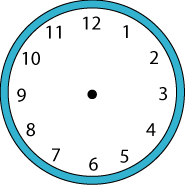#### You may also like### Prompt Cards

These two group activities use mathematical reasoning - one is numerical, one geometric.### Consecutive Numbers

An investigation involving adding and subtracting sets of consecutive numbers. Lots to find out, lots to explore.### Exploring Wild & Wonderful Number Patterns

EWWNP means Exploring Wild and Wonderful Number Patterns Created by Yourself! Investigate what happens if we create number patterns using some simple rules.

# Clock Face

##### Age 7 to 11 Challenge Level:

Can you draw a straight line across the centre of a clock face so that the numbers on both sides of the line have the same total?Can you do this in another way?

Can you draw two lines (like the hands of the clock) to divide the clock face so that the total of the numbers on one side of the lines is twice the total on the other side? Can you do this in another way?

Can you divide the clock face so that that the total on one side of the lines is five times more than the total on the other side? Can you do this in another way?

Can you draw two lines to divide the numbers so that the total of the numbers on each side of the lines are both multiples of six? In how many different ways can you do this?

Can you draw two lines so that the numbers on each side add to a prime number? Can you do this in another way?

Can you find any other interesting ways to group the numbers on a clock face by drawing two lines?

You might find this sheet useful for working on.